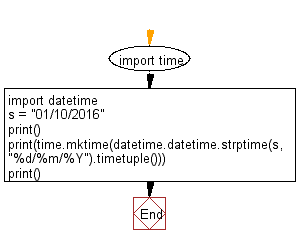﻿ Python: Convert a string date to the timestamp - w3resource

# Python: Convert a string date to the timestamp

## Python Datetime: Exercise-31 with Solution

Write a Python program to convert a string date to a timestamp.

Sample Solution:

Python Code:

``````import time
import datetime
s = "01/10/2016"
print()
print(time.mktime(datetime.datetime.strptime(s, "%d/%m/%Y").timetuple()))
print()
```
```

Sample Output:

```1494225605.0
```

Flowchart:Python Code Editor: